Integration Full Chapter Explained - Integration Class 12 - Everything you need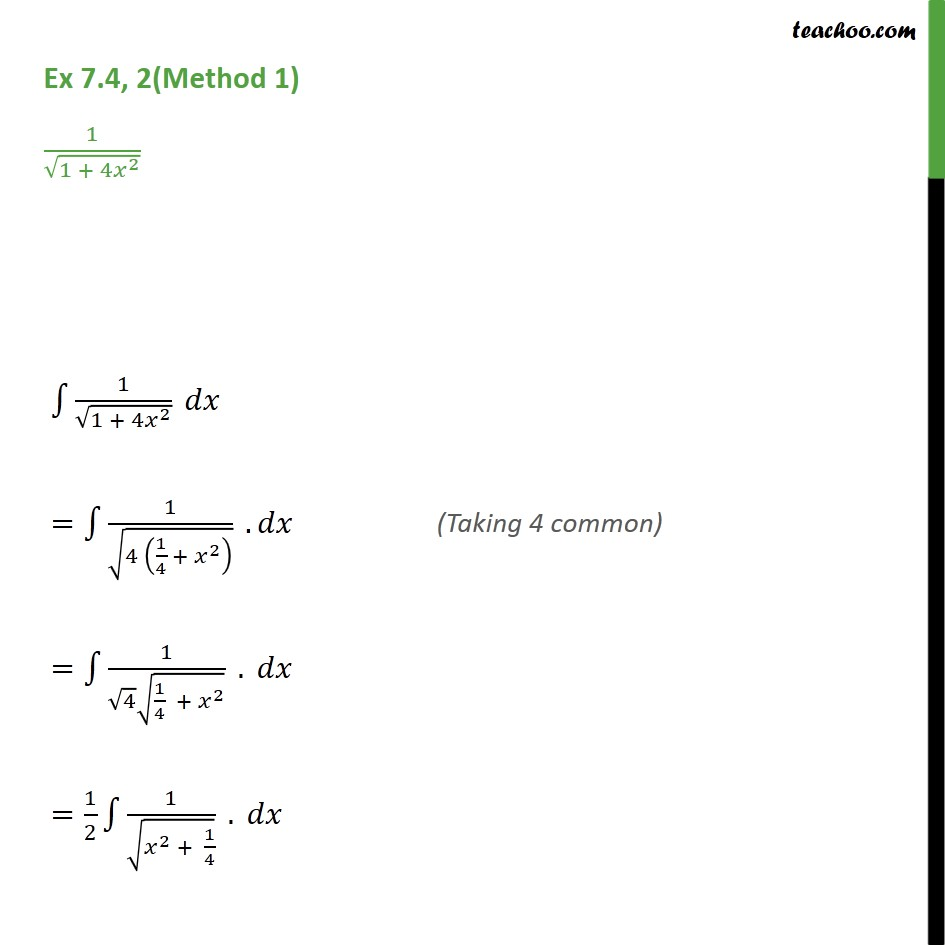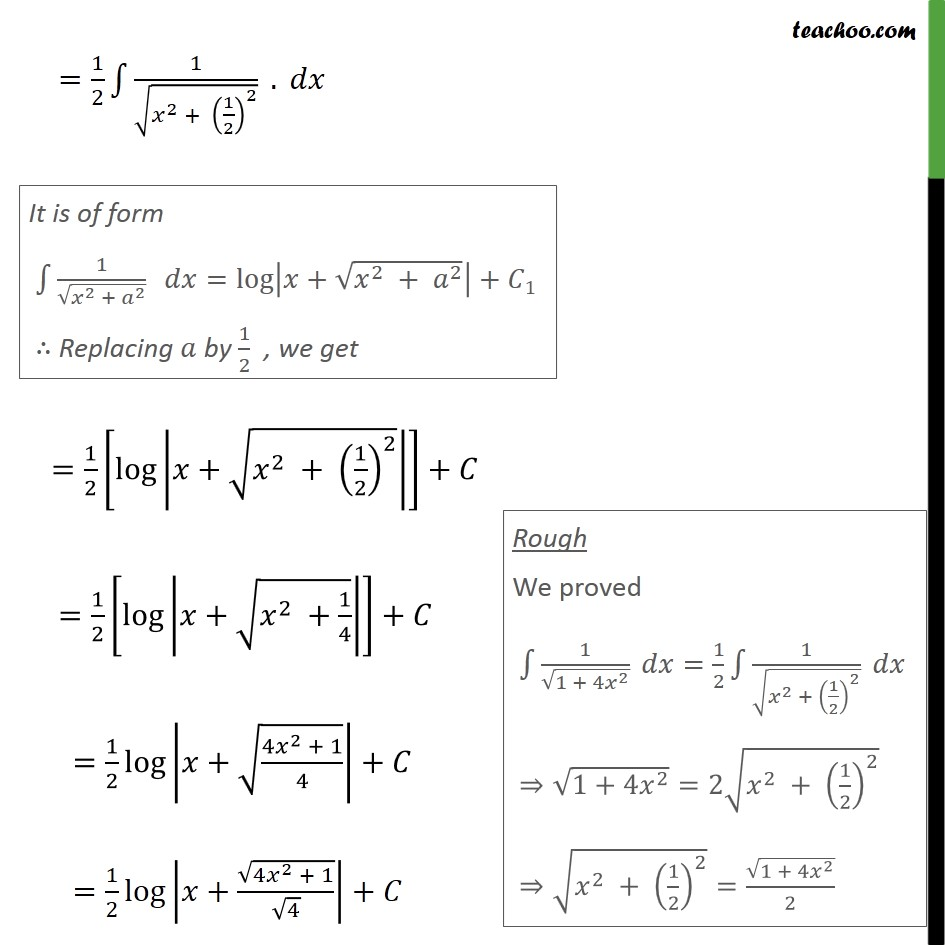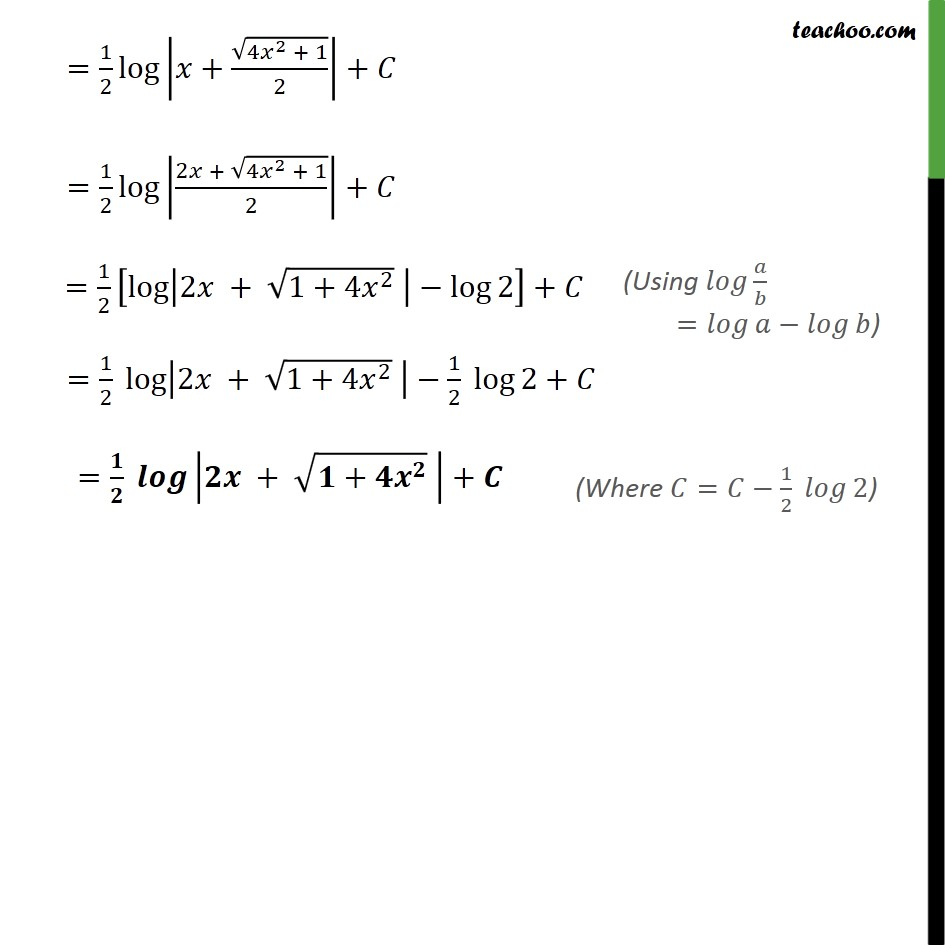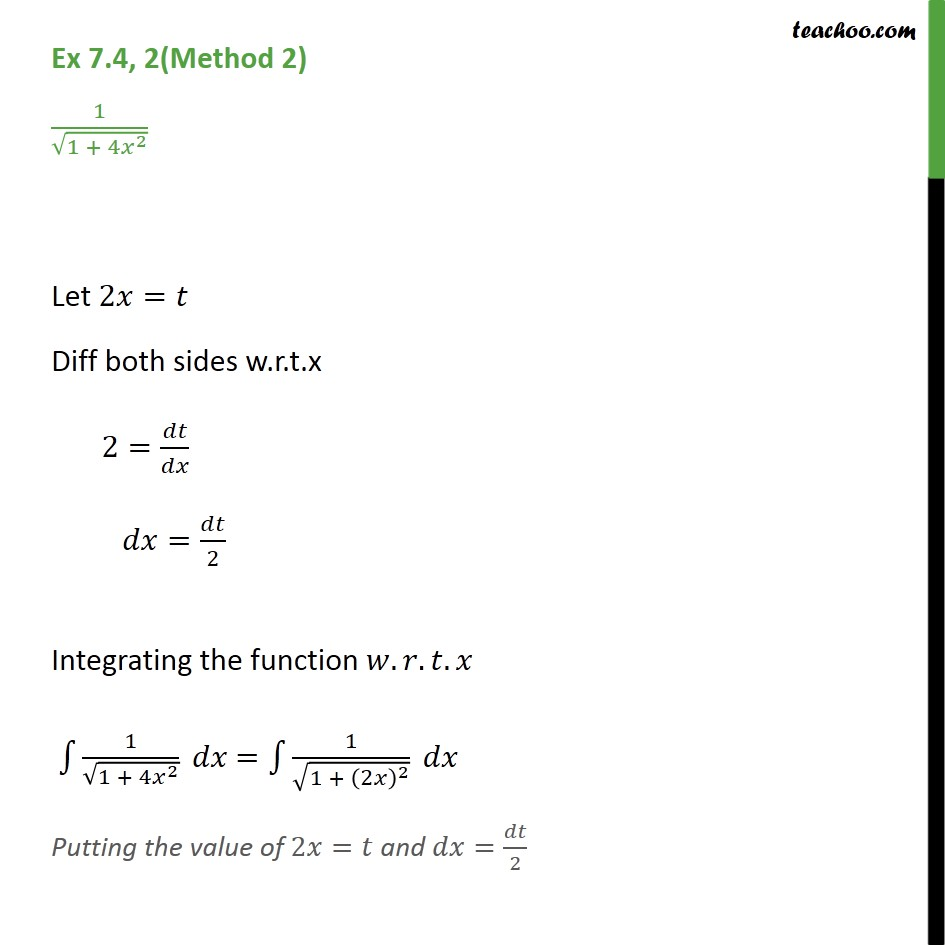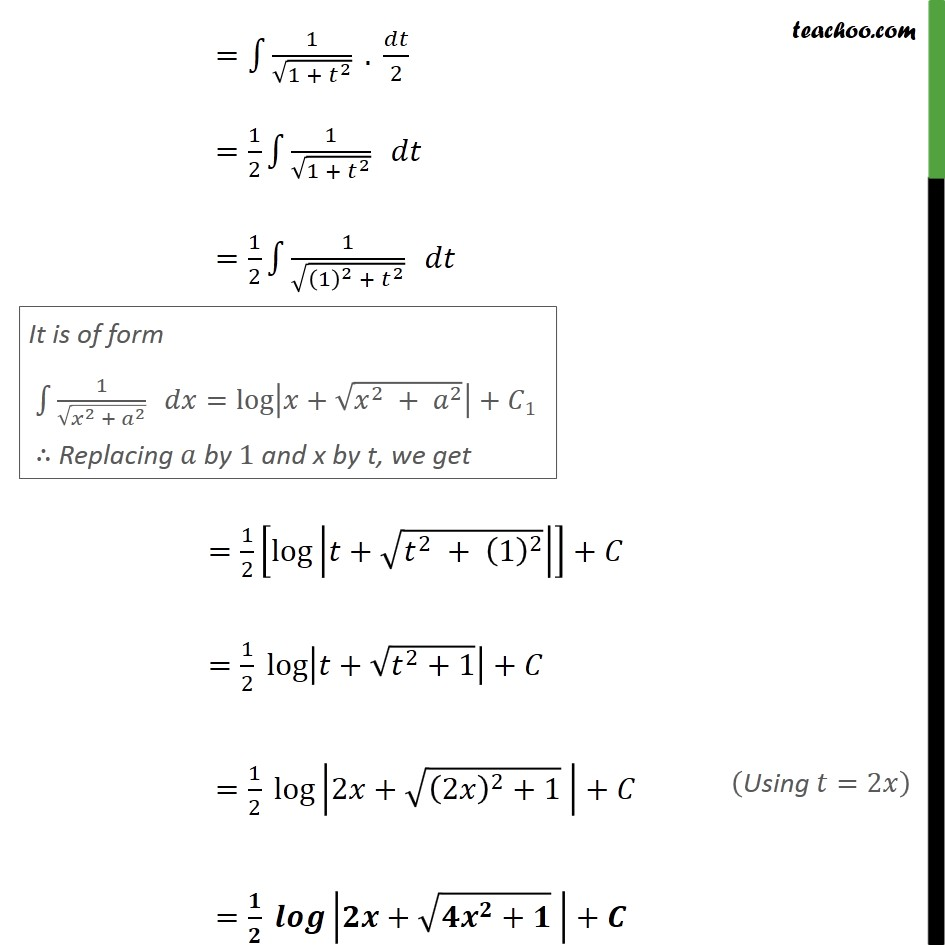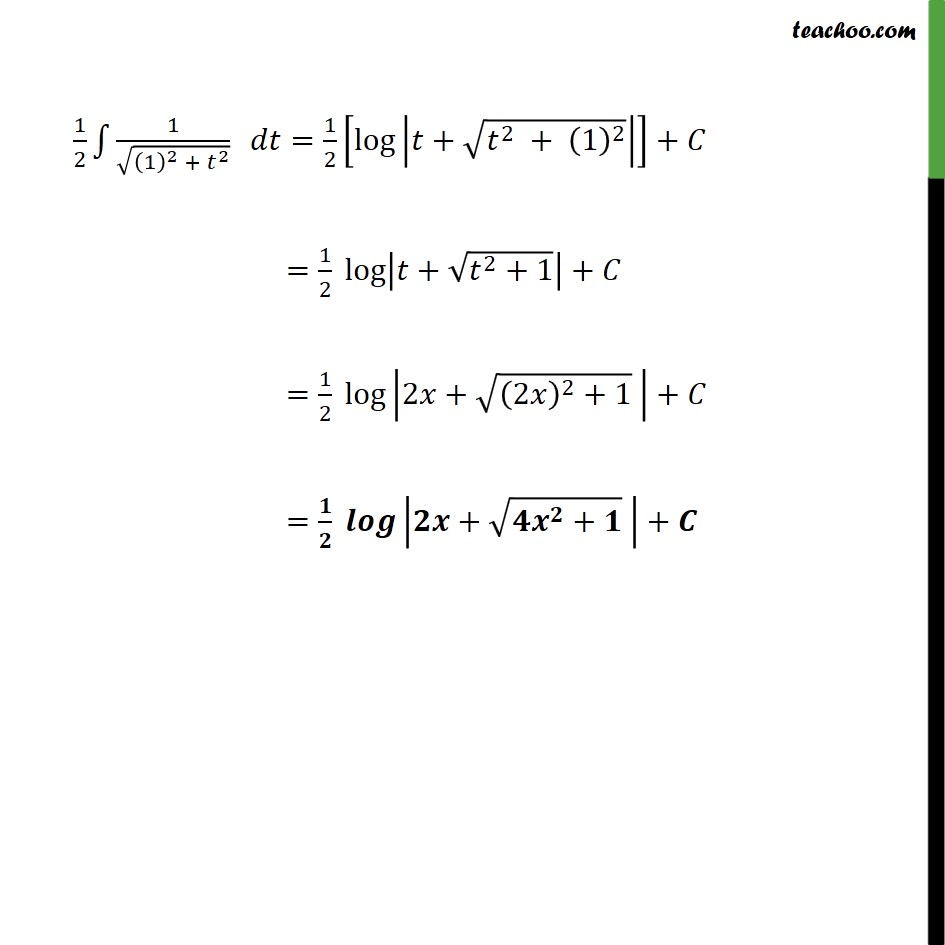1. Chapter 7 Class 12 Integrals
2. Serial order wise
3. Ex 7.4

Transcript

Ex 7.4, 2(Method 1) 1/√(1 + 4𝑥^2 ) ∫1▒1/√(1 + 4𝑥^2 ) 𝑑𝑥 =∫1▒1/√(4 (1/4 + 𝑥^2 ) ) . 𝑑𝑥 =∫1▒1/(√4 √(1/4 + 𝑥^2 )) . 𝑑𝑥 =1/2 ∫1▒1/√(𝑥^2 + 1/4) . 𝑑𝑥 =1/2 log⁡|𝑥+√(4𝑥^2 + 1)/2|+𝐶 =1/2 log⁡|(2𝑥 + √(4𝑥^2 + 1))/2|+𝐶 =1/2 [log⁡|2𝑥 + √(1+4𝑥^2 ) |−log⁡2 ]+𝐶 =1/2 log⁡|2𝑥 + √(1+4𝑥^2 ) |−1/2 log⁡2+𝐶 =𝟏/𝟐 𝒍𝒐𝒈⁡|𝟐𝒙 + √(𝟏+𝟒𝒙^𝟐 ) |+𝑪

Ex 7.4

Chapter 7 Class 12 Integrals
Serial order wise

About the AuthorDavneet Singh
Davneet Singh is a graduate from Indian Institute of Technology, Kanpur. He has been teaching from the past 9 years. He provides courses for Maths and Science at Teachoo.